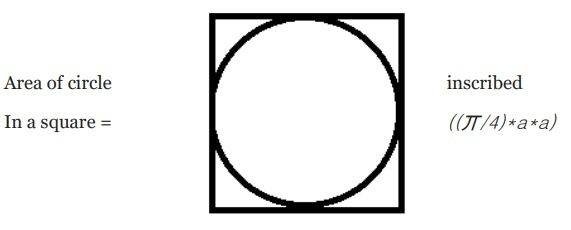# Program to calculate the area of an Circle inscribed in a Square

A circle inscribed in a square is a circle which touches the sides of the circle at its ends. I.e. the diameter of the inscribed circle is equal to the side of the square. The area can be calculated using the formula “((丌/4)*a*a)” where ‘a’ is the length of side of square.Logic of the Code - The area of circle inscribed inside the circle is calculated using the formula ((丌/4)*a*a) for this we need to define the value of pie (丌) that mathematically is 22/7 or 3.14. The expression that evaluates to the result is saved in a float variable.

## Example

Live Demo

#include <stdio.h>
#include <math.h>
int main() {
int a = 5;
float area;
float pie = 3.14;
printf("Program to find area of circle inscribed inside a square");
printf("The side of the square is %d ", a);
area = ((pie/4)*a*a);
printf("The area of circle inscribed inside a square is %f ", area);
return 0;
}

## Output

The side of the square is 5
The area of circle inscribed inside a square is 19.625000